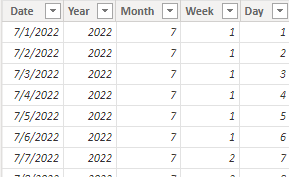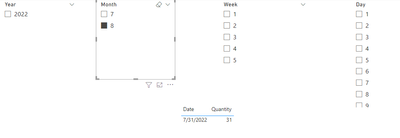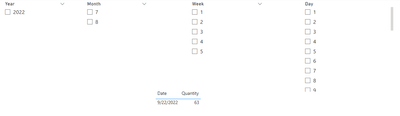cancel
Showing results for
Did you mean:Frequent Visitor

## Display value based on Month, Week and Day filters selectionI have 4 filters coming from same calendar table. Year, Month, Weeknumber of the month and day

Requirement:

1. When August month is selected, quantity should display value of last day of pervious month i.e July
2. When Weeknum filter is selected, quantity should display value for last day of the selected week
3. When day is selected, quantity should display value of the selected day
4. If no filter is selected, by default quantity should display value of current day

Note: Quantity is a direct column coming from Product table.
Please let me know on how to approach this scenario

2 ACCEPTED SOLUTIONSSuper User

@kruthikav , try measure like. Assumes year is selected and all date related values are coming from date table

var _max = if(isfiltered('Date'),MAX( 'Date'[Date]) , today())
var _weekend = _max+ 7-1*WEEKDAY(_max,2)
return
Switch(True(),
isfiltered(Date[month]), calculate([Measure],,DATESBETWEEN('Date'[Date],eomonth(_max,-1),eomonth(_max,-1))),
isfiltered(Date[month]), calculate([Measure],,DATESBETWEEN('Date'[Date],_weekend,_weekend)),
isfiltered(Date[day]), calculate([Measure],,DATESBETWEEN('Date'[Date],_max,_max)),
calculate([Measure],,DATESBETWEEN('Date'[Date],today(),today())))

https://powerpivotpro.com/2013/03/hasonevalue-vs-isfiltered-vs-hasonefilter/Community Support

Hi @kruthikav ,

According to your description, I create a sample.

Product table:Calendar table:Here's my solution.

Don't make relationship between the two tables, create a measure:

``````Check =
SWITCH (
TRUE (),
ISFILTERED ( 'Calendar'[Month] )
&& MAX ( 'Product'[Date] )
= EOMONTH ( DATE ( 2022, SELECTEDVALUE ( 'Calendar'[Month] ), 1 ), -1 ), 1,
ISFILTERED ( 'Calendar'[Week] )
&& LOOKUPVALUE ( 'Calendar'[Week], 'Calendar'[Date], MAX ( 'Product'[Date] ) + 1 )
= SELECTEDVALUE ( 'Calendar'[Week] ) + 1
&& LOOKUPVALUE ( 'Calendar'[Week], 'Calendar'[Date], MAX ( 'Product'[Date] ) )
= SELECTEDVALUE ( 'Calendar'[Week] ), 1,
ISFILTERED ( 'Calendar'[Day] )
&& DAY ( MAX ( 'Product'[Date] ) ) = SELECTEDVALUE ( 'Calendar'[Day] ), 1,
NOT ( ISFILTERED ( 'Calendar' ) )
&& MAX ( 'Product'[Date] ) = TODAY (), 1,
0
)
``````

Put the measure in the visual filter and select its value to 1.Get the result:I attach my sample below for your reference.

Best Regards,
Community Support Team _ kalyj

If this post helps, then please consider Accept it as the solution to help the other members find it more quickly.

3 REPLIES 3Community Support

Hi @kruthikav ,

According to your description, I create a sample.

Product table:Calendar table:Here's my solution.

Don't make relationship between the two tables, create a measure:

``````Check =
SWITCH (
TRUE (),
ISFILTERED ( 'Calendar'[Month] )
&& MAX ( 'Product'[Date] )
= EOMONTH ( DATE ( 2022, SELECTEDVALUE ( 'Calendar'[Month] ), 1 ), -1 ), 1,
ISFILTERED ( 'Calendar'[Week] )
&& LOOKUPVALUE ( 'Calendar'[Week], 'Calendar'[Date], MAX ( 'Product'[Date] ) + 1 )
= SELECTEDVALUE ( 'Calendar'[Week] ) + 1
&& LOOKUPVALUE ( 'Calendar'[Week], 'Calendar'[Date], MAX ( 'Product'[Date] ) )
= SELECTEDVALUE ( 'Calendar'[Week] ), 1,
ISFILTERED ( 'Calendar'[Day] )
&& DAY ( MAX ( 'Product'[Date] ) ) = SELECTEDVALUE ( 'Calendar'[Day] ), 1,
NOT ( ISFILTERED ( 'Calendar' ) )
&& MAX ( 'Product'[Date] ) = TODAY (), 1,
0
)
``````

Put the measure in the visual filter and select its value to 1.Get the result:I attach my sample below for your reference.

Best Regards,
Community Support Team _ kalyj

If this post helps, then please consider Accept it as the solution to help the other members find it more quickly.Super User

@kruthikav , try measure like. Assumes year is selected and all date related values are coming from date table

var _max = if(isfiltered('Date'),MAX( 'Date'[Date]) , today())
var _weekend = _max+ 7-1*WEEKDAY(_max,2)
return
Switch(True(),
isfiltered(Date[month]), calculate([Measure],,DATESBETWEEN('Date'[Date],eomonth(_max,-1),eomonth(_max,-1))),
isfiltered(Date[month]), calculate([Measure],,DATESBETWEEN('Date'[Date],_weekend,_weekend)),
isfiltered(Date[day]), calculate([Measure],,DATESBETWEEN('Date'[Date],_max,_max)),
calculate([Measure],,DATESBETWEEN('Date'[Date],today(),today())))

https://powerpivotpro.com/2013/03/hasonevalue-vs-isfiltered-vs-hasonefilter/Frequent Visitor

Above DAX is working only for Month filter. Values are not getting changed for week and day filter.